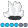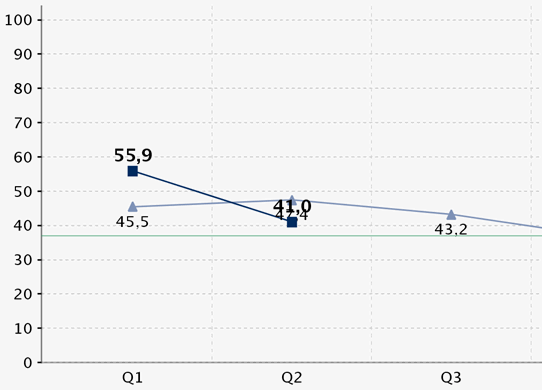Eva
Quartz | Level 8

## gplot: sum on symbol like sum on bar in gchart possible?

Dear all,

I have a plot with connected symbols. Is it possible to write the vaxis value on top of each symbol like in this example?I know it is possible with prog gchart... vbar. You simply write sum into the vbar statement. I tried it with the plot statement but get the error that the syntax is not correct.

Best wishes

Eva

1 ACCEPTED SOLUTION

Accepted Solutions

## Re: gplot: sum on symbol like sum on bar in gchart possible?

You can use Proc Gplot's "pointlabel" for that.  By default, it uses the y-value as the label (which is what you want, I believe?).  You can also customize the label, and use another variable if you want.

symbol1 value=dot interpol=none pointlabel;

proc gplot data=sashelp.class;

plot height*weight=1;

run;

6 REPLIES 6

## Re: gplot: sum on symbol like sum on bar in gchart possible?

You can use Proc Gplot's "pointlabel" for that.  By default, it uses the y-value as the label (which is what you want, I believe?).  You can also customize the label, and use another variable if you want.

symbol1 value=dot interpol=none pointlabel;

proc gplot data=sashelp.class;

plot height*weight=1;

run;Eva
Quartz | Level 8

## Re: gplot: sum on symbol like sum on bar in gchart possible?

Dear Robert,

thanx. I would never have googled for "pointlabel" 🙂 It works. And foundit now in the sas help.

Best wishes

EvaEva
Quartz | Level 8

## Re: gplot: sum on symbol like sum on bar in gchart possible?

I formatted the variable I user for the pointlabel now in commax10.2

Unfortunately then the values on the y-axis are also in this format. Can I use a different format for the values on an axis?

## Re: gplot: sum on symbol like sum on bar in gchart possible?

That's where the "You can also customize the label, and use another variable if you want" comes in, such as ...

data my_data; set sashelp.class;

my_text=put(height,commax10.2);

run;

symbol1 value=dot interpol=none pointlabel=('#my_text');

proc gplot data=my_data;

plot height*weight=1;

run;Eva
Quartz | Level 8

## Re: gplot: sum on symbol like sum on bar in gchart possible?

Unfortunately not supported by activex. Like axis split='...' is not 😞

## Re: gplot: sum on symbol like sum on bar in gchart possible?

Ahh - you hadn't mentioned you were using Activex!

A lot of little things aren't supported in dev=Activex (and dev=Java), and you are quite likely to bump into these when creating custom graphs.  Therefore, I always recommend that people creating custom graphs use dev=png.

Discussion stats
• 6 replies
• 789 views
• 0 likes
• 2 in conversation